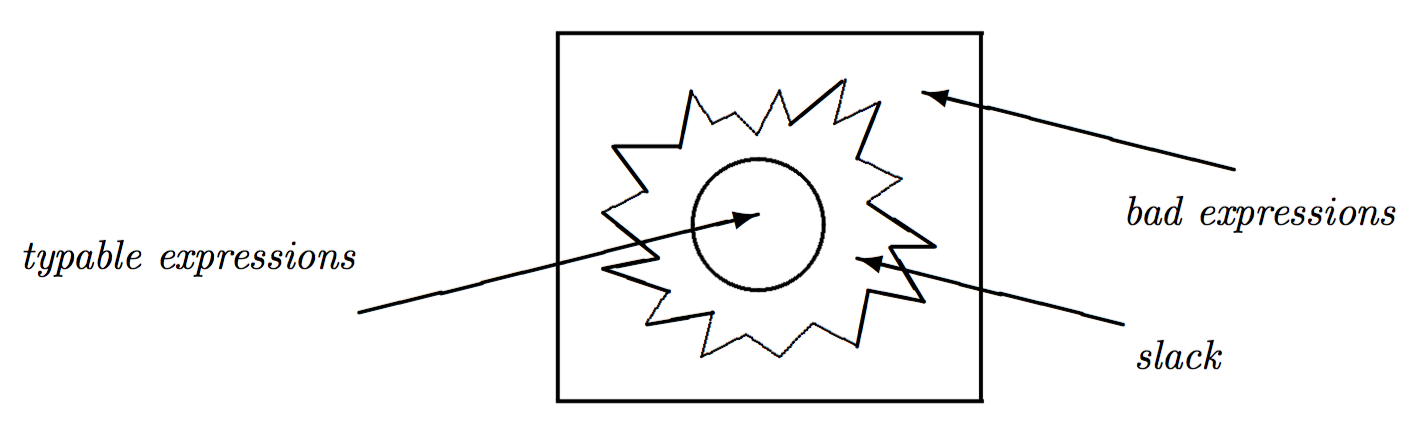@darwin-yuan 2016-07-03T01:56:21.000000Z 字数 5998 阅读 6156

# Hindley-Milner类型系统（1）

类型系统 HaskellHindley-Milner类型系统，又称Hindley-Domas-Milner类型系统。目标是在没有任何程序员给出类型注解的情况下，可以自动推导出任意表达式的类型。

## 语法构造

1. 变量：$x$
2. 函数调用：$e_{1}\ e_{2}$
3. 函数定义：$\lambda x.e$
4. let绑定：$\mathbf{let}\ x = e_{1}\ \mathbf{in}\ e_{2}$

## 类型

HM类型系统定义里，$\tau$被称做类型Type），它可以是一个：

• 原生类型$\iota$
• 类型变量$\alpha$
• 函数类型$\tau \rightarrow \tau$

$\sigma$ 被称做type scheme。它即可以是一个 $\tau$，即type；也可以是一个$\forall \alpha.\sigma$。而后者，不是type

id :: forall a. a -> aid x = x

id_Int :: Int -> Intid_Int x = xid_Bool :: Bool -> Boolid_Bool x = xid_F :: (Int -> Bool) -> (Int -> Bool)id_F x = x-- a lot more others ...

$\forall$ 可被看作类型世界的 $\lambda$

## PolyType & MonoType

• $\forall \alpha. \sigma$，之前没有名字，现在被称为多态类型polytype）；
• 相应的，$\tau\$，之前我们称做type，现在改称为单态类型monotype）；
• 然后，我们就可以把type scheme称做类型type）。

monotype，其中monomonomorphism的缩写，指单态映射。因而，monotype被称做单态类型。很容易理解，如下类型都属于monotype

f :: (a -> b) -> a -> bf g x = g x

## 不变的变量

$\lambda$的世界里，操作的是数值Data Value）。比如1, 2, 0, True, False。这些值，一般被称做literal constant

f :: forall a b. (a -> b) -> a -> bf g x = g x

f的类型是一个polytype，但其中的任何类型变量都是monotype。虽然 $a$ 在类型 $\forall a\ b. (a \rightarrow b) \rightarrow a \rightarrow b$ 里出现了两次，但它们所映射的类型必然相同。变量 $b$ 也一样。

HM类型系统里，所有的类型变量都是单态类型monotype）。

## $\lambda$ 和 $\Lambda$

Simple-Typed Lambda系统是不需要 $\Lambda$ 的，在那个系统里，类型只被当作数据的一个属性。但是，在更加高阶的polymorphic type lambda系统里，就需要 $\Lambda$ 当作计算类型的函数，类型是其参数和结果。

$\lambda$ 一样，$\Lambda$ 也具备 $\alpha$ 变换，$\beta$ 规约，以及 $\eta$ 变换。比如：$\Lambda \alpha.\alpha \rightarrow\alpha$$\Lambda\beta.\beta\rightarrow\beta$ 完全等价；而 $(\Lambda\alpha.\alpha\rightarrow\alpha)\ \beta=\beta\rightarrow\beta$

$\lambda$ $\Lambda$
Value monotype
Literal Constant: 0,1,2,True,False Primitive: Int,Bool
Variable: $x,y$ Type Variable: $\alpha,\beta$
Variable Binding Type Variable Binding
Immutable Variable Immutable Type Variable
Function Application Type Instantiation
Type Kind

$\lambda$演算里，所有的变量都是mono-value，即一个变量和数值之间只能存在一个唯一的映射，一旦绑定，不可变更。

(*) :: Int -> Int -> Int(*) = \x y -> x valMul y(+) :: Int -> Int -> Int(+) = \x y -> x valPlus y

$(,)$则实现了类型系统的乘法：

Either是一个type lambda，而Either Int Bool则是一个从type lambda计算得到的type。同时，Either是一个polytype，而Either Int Bool是一个monotype。因而polytype就是type lambda• 私有
• 公开
• 删除